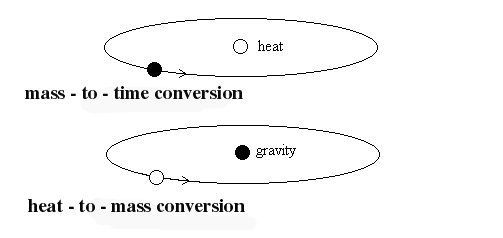﻿ time-to-heat transformation
Heat-to-Time and Time-to-Heat Conversion
Alexander V. Frolov

According to N.Kozyrev's theory , there is no thermonuclear energy source in stars but any star is special machine that produce heat energy from incoming energy flow. This input energy, by N.Kozyrev is so called "time flow energy". In other words, it is gravitation field energy.

Other authors described possible relations and transformations between electromagnetic form of energy and gravitation form of energy.

If the Sun is transformer of some kind energy into the heat, there is opposite way also. Let's assume some heat energy source is rotating one. In center of this rotation there is the possibility for gravitation (time) energy collection. Also I have to note that linear motion of heat source is the way to produce the field also.

Fig.1 show the rotation of mass (point gravity charge) and rotation of heat source (point heat charge) are two opposite processes. There is an analogy with magnetic field that is produced by any motion of electric charge, both linear of curved motion. In closed trajectory of electric coil the field can be concentrated in center of the trajectory.  By this way gravitation field can be concentrated also. Detector that is placed in this point should demonstrate some changes in space-time structure.Fig1.

I produce some simple experiments to test the idea in July 1999. Rotation of 2 Watt power heat source was organized for R=0.07 m and velocity about 300 rotation per second. Axial field was detected by special electromagnetic equipment since the field produce changes of inner structure of the detector element, for example, its resistance.

Possible problems in reproduction of this experiment are connected with biological activity of the generated field.

References